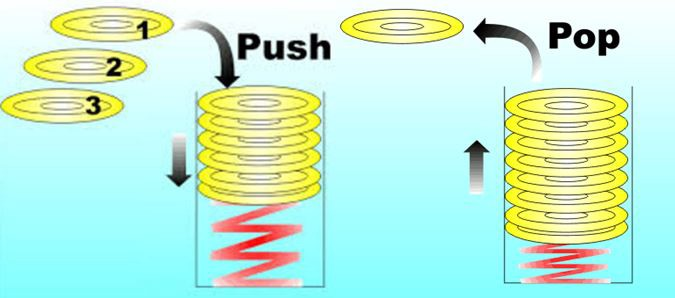# Solidity汇编ezpod 2020-02-16

## 2、以太坊虚拟机和堆栈结构机器

``````contract Assembler {
function do_something_cpu() public {
assembly {
// start writing evm assembler language
}
}
}``````

EVM是一个栈虚拟机，栈这种数据结构只允许两个操作：压入（PUSH）或弹出（POP）数据。最后压入的数据位于栈顶，因此将被第一个弹出，这被称为后进先出（LIFO：Last In, First Out）：## 3、堆栈结构机器的操作码

``````a + b      // 标准表示法Infix

## 4、在Solidity合约中使用内联汇编

``````assembly {
// some assembly code here
}``````

`assembly`块内的代码开发语言被称为Yul，为了简化我们称其为汇编或EVM汇编。

``````assembly {
let x := 2
}
assembly {
let y := x          // Error
}``````

``````// DeclarationError: identifier not found
// let y := x
// ^``````

``````function addition(uint x, uint y) public pure returns (uint) {
assembly {
let result := add(x, y)   // x + y
mstore(0x0, result)       // 在内存中保存结果
return(0x0, 32)           // 从内存中返回32字节
}
}``````

``````function addition(uint x, uint y) public pure returns (uint) {
assembly {
// 创建一个新的变量result
//     -> 将计算结果赋值给变量result
let result := add(x, y)   // x + y

// 使用mstore操作码
//     -> 将result变量的值存入内存
//     -> 指定内存地址 0x0
mstore(0x0, result)       // 将结果存入内存

// 从内存地址0x返回32字节
return(0x0, 32)
}
}``````

## 5、Solidity汇编中的变量定义与赋值

``````assembly {
let x := 2
}``````

``````assembly {
let x           // 自动初始化为 x = 0
x := 5          // x 现在的值是5
}``````

``````assembly {
let x := 7
let z := add(keccak256(0x0, 0x20), div(slength, 32))
let n
}``````

## 6、Solidity汇编中let指令的运行机制

• 创建一个新的堆栈槽位
• 为变量保留该槽位
• 当到达代码块结束时自动销毁该槽位

## 7、Solidity汇编中的注释

``````assembly {
// single line comment

/*
Multi
line
comment
*/
}``````

## 8、Solidity汇编中的字面量

``````assembly {
let a := 0x123             // 16进制
let b := 42                // 10进制
let c := "hello world"     // 字符串

let d := "very long string more than 32 bytes" // 超长字符串，错误！
} ``````

## 9、Solidity汇编中的块和作用范围

``````assembly {
let x := 3          // x在各处可见

// Scope 1
{
let y := x     // ok
}  // 到此处会销毁y

// Scope 2
{
let z := y     // Error
} // 到此处会销毁z
}

// let z := y
// ^``````

## 10、在Solidity汇编中使用函数的局部变量

``````function assembly_local_var_access() public pure {
uint b = 5;
assembly {                // defined inside  an assembly block
let y := 10
}
assembly {               // defined outside an assembly block
let y := mul(x, b)
}
}``````

## 11、在Solidity汇编中使用for循环

``````function for_loop_solidity(uint n, uint value) public pure returns(uint) {
for ( uint i = 0; i < n; i++ ) {
value = 2 * value;
}
return value;
}``````

``````function for_loop_assembly(uint n, uint value) public pure returns (uint) {
assembly {
for { let i := 0 } lt(i, n) { i := add(i, 1) } {
value := mul(2, value)
}
mstore(0x0, value)
return(0x0, 32)
}
}``````

• 初始化：`let i := 0`
• 执行条件：`lt(i, n)` ，必须是函数风格表达式
• 迭代后续步骤：`add(i, 1)`

## 12、在Solidity汇编中使用while循环

``````assembly {
let x := 0
let i := 0
for { } lt(i, 0x100) { } {     // 等价于：while(i < 0x100)
}
}``````

## 13、在Solidity汇编中使用if语句

Solidity内联汇编支持使用`if`语句来设置代码执行的条件，但是没有其他语言中的`else`部分。

``````assembly {
if slt(x, 0) { x := sub(0, x) }  // Ok
if eq(value, 0) revert(0, 0)     // Error, 需要大括号
}``````

if语句强制要求代码块使用大括号，即使需要保护的代码只有一行，也需要使用大括号。这和solidity不同。

## 14、在Solidity汇编中使用switch语句

EVM汇编中也有`switch`语句，它将一个表达式的值于多个常量进行对比，并选择相应的代码分支来执行。`switch`语句支持一个默认分支`default`，当表达式的值不匹配任何其他分支条件时，将执行默认分支的代码。

``````assembly {
let x := 0
case 0 {
}
default {
}
sstore(0, div(x, 2))
}``````

`switch`语句有一些限制：

• 分支列表不需要大括号，但是分支的代码块需要大括号- 所有的分支条件值必须：1）具有相同的类型 2）具有不同的值- 如果分支条件已经涵盖所有可能的值，那么不允许再出现default条件
``````assembly {
let x := 34

switch lt(x, 30)
case true {
// do something
}
case false {
// do something els
}
default {
// 不允许
}
}``````

## 15、在Solidity汇编中使用函数

``````assembly {
function allocate(length) -> pos {
}
let free_memory_pointer := allocate(64)
}``````

• 从堆栈提取参数
• 将结果压入堆栈

## 16、Solidity汇编中的操作码

EVM操作码可以分为以下几类：

• 算数和比较操作
• 位操作
• 密码学操作，目前仅包含`keccak256`
• 环境操作，主要指与区块链相关的全局信息，例如`blockhash``coinbase`收款账号
• 存储、内存和栈操作
• 交易与合约调用操作
• 停机操作
• 日志操作

1591人浏览
2020-04-07

solidity语言介绍以及开发环境准备
844人浏览
2018-05-27 22:41:00
【Solidity】使用编译器
893人浏览
2017-09-20 10:34:00
【Solidity】8. 杂项 - 深入理解Solidity
1213人浏览
2017-09-18 10:14:00
solidity 0.5.7 快速入门教程
817人浏览
2019-04-27 21:04:13

1100人浏览
2017-09-05 07:56:41

1885人浏览
2018-01-21 16:05:00

933人浏览
2018-04-30 20:50:47
solidity_mapping_implementation
718人浏览
2018-07-01 11:42:00
Solidity编辑器Remix中文版
866人浏览
2019-04-29 01:29:27
0
0
196